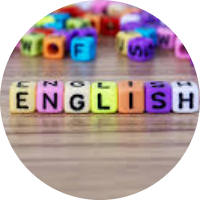# Lines and Angles

This set of MCQs helps you brush up on important math topics and prepare you to dive into skill practice.

Start Quiz

What does the image show?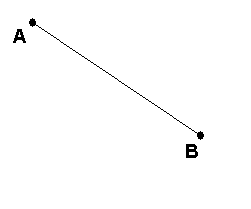A line

A ray

A line segment

A point

What does this image show?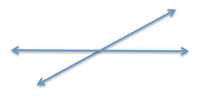Line segment

Perpendicular lines

Parallel lines

Intersecting lines

Find the value of x?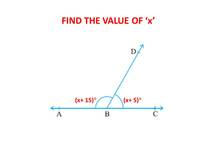70

60

120

80

An angle which is the complement of itself is_

45

90

30

60

What is the value of x?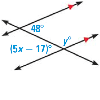12

13

6.5

7

If AB || CD, EF ⊥ CD, and ∠GED = 135° as per the figure given below. The value of ∠AGE is: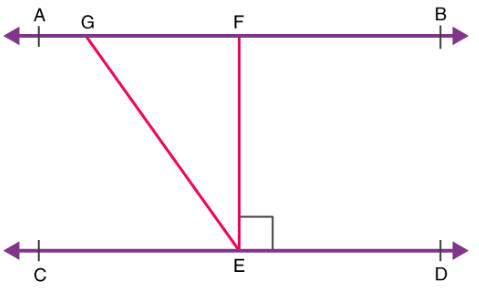90

140

135

45

Two parallel lines intersect at:

One point

Two point

Three point

Null

In the figure below, which of the following are corresponding angle pairs?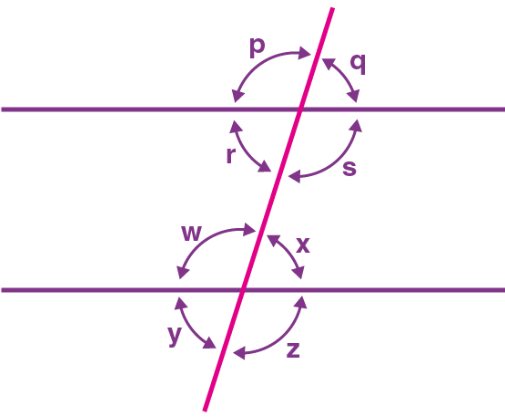P and Q

P and W

P and X

P and Z

Quiz/Test Summary
Title: Lines and Angles
Questions: 8
Contributed by: